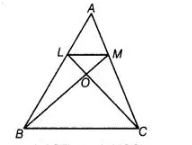# In ΔABC, if L and M are the points on AB and AC,`
Question:

In ΔABC, if L and M are the points on AB and AC, respectively such that LM || BC. Prove that ar (ΔLOB) = ar (ΔMOC).

Solution:

Given In ΔABC, L and M are points on AB and AC respectively such that LM || BC.To prove $\operatorname{ar}(\Delta \mathrm{LOB})=\operatorname{ar}(\triangle \mathrm{MOC})$

Proof We know that, triangles on the same base and between the same parallels are equal in area.

Hence, $\triangle B C$ and $\triangle M B C$ lie on the same base $B C$ and between the same parallels $B C$ and $L M$.

So, $\operatorname{ar}(\Delta B C)=\operatorname{ar}(\Delta M B C)$

$\Rightarrow \quad \operatorname{ar}(\Delta L O B)+\operatorname{ar}(\triangle B O C)=\operatorname{ar}(\Delta M O C)+\operatorname{ar}(\Delta B O C)$

On eliminating $D$ ar ( $\triangle B O C$ ) from both sides, we get

$\operatorname{ar}(\triangle \perp O B)=\operatorname{ar}(\triangle M O C)$

Hence proved.